# What is Declaration Merging in Typescript ?

• Last Updated : 16 Mar, 2022

In Typescript, the term “declaration merging” refers to the compiler combining two declarations with the same name into a single definition. Both of the initial declarations are present in this combined definition. It is possible to merge Interfaces, namespaces and enums and so on but classes cannot be merged.

Interface with Interface Merging: In this example, 3 interfaces are declared with the same name ‘Student’, so all the interfaces are merged. class Student1 implements the merged Interface, it has the properties of all three interfaces.

## Javascript

 `interface Student { ` `    ``id: number; ` `} ` ` `  `interface Student { ` `    ``name: string; ` `} ` ` `  `interface Student { ` `    ``branch: string; ` `} ` ` `  `class Student1 implements Student { ` `    ``id = 56545; ` `    ``name = ``"sarah"``; ` `    ``branch = ``"CSE"``; ` `} ` ` `  `const student = ``new` `Student1(); ` `console.log(student);`

Output:

`Student1 { id: 56545, name: 'sarah', branch: 'CSE' }`

In this example, the same property name is repeated in interfaces, but the datatype is changed. in the first interface property id has type ‘number’, in the second interface it has type ‘string’. Typescript compiler raises error saying “Property ‘id’ must be of type ‘number’, but here has type ‘string'”. here properties do not refer to ‘functions’.

## Javascript

 `interface Student { ` `    ``id: number; ` `} ` ` `  `interface Student { ` `    ``// error: Subsequent property declarations ` `    ``// must have the same type.  ` `    ``// Property 'id' must be of type 'number',  ` `    ``// but here has type 'string'. ` `    ``id: string; ` `    ``name: string; ` `} ` ` `  `interface  Student { ` `    ``branch: string; ` `}`

Output:

```error TS2717: Subsequent property declarations must have the same type.
Property 'id' must be of type 'number', but here has type 'string'.
id: string;
~~
two.ts:252:3
id: number;
~~
'id' was also declared here.```

What if Interfaces have functions declared with the same name but the types of the arguments are different? When the components in the merged interfaces are functions with the same name, they are overloaded, which means that the relevant function will be called based on the kind of input given.

## Javascript

 `interface Student { ` `    ``displayId(id: number); ` `} ` ` `  `interface Student { ` `    ``displayId(id: string); ` `} ` ` `  `const student: Student = { ` `    ``displayId(id) { ` `        ``return` `id; ` `    ``}, ` `}; ` ` `  `console.log(student.displayId(``"AD54"``)); ` `console.log(student.displayId(54));`

Output:

```AD54
54```

When interfaces with the same signature are combined or merged, the functions which are declared in the recently created interfaces appear at the top of the merged interface, while the functions from the previously created interfaces appear below.

## Javascript

 `interface Student { ` `  ``displayId(id: number); ` `} ` ` `  `interface Student { ` `  ``displayId(id: string); ` `}` `# precedence in the merged interface  ` `# merged interface ` `interface Student { ` `  ``displayId(id: string); ` `  ``displayId(id: number); ` `}`

Output: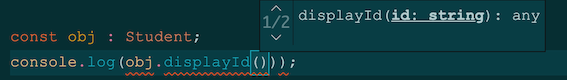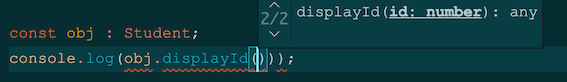With the rest of the theory being the same, there’s one exception. functions with string literal types are given greater precedence and hence appear first.

## Javascript

 `interface Student { ` `    ``displayId(id: number); ` `} ` ` `  `interface Student { ` `    ``displayId(id: string); ` `    ``displayId(id: ``"AC612"``); ` `} ` `interface Student { ` `    ``displayId(id: ``"AD645"``); ` `}` ` `  `# Precedence in the merged interface  ` `# merged interface ` `interface Student{ ` `    ``displayId(id: ``"AC612"``); ` `    ``displayId(id: ``"AD645"``); ` `    ``displayId(id: string); ` `    ``displayId(id: number); ` `}`

Output: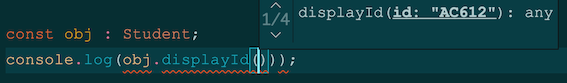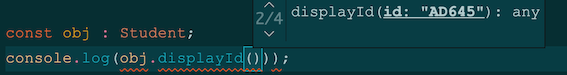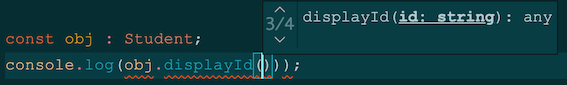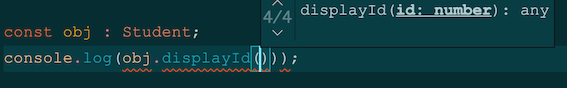My Personal Notes arrow_drop_up
Recommended Articles
Page :